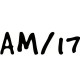# CBSE Class 12 Maths Notes Continuity and DifferentiabilityWritten by

Continuity and Differentiability is part of Class 12 Maths Notes for Quick Revision. Here we have given Class 12 Maths Notes Continuity and Differentiability.Continuity and Differentiability

## Continuity at a Point

A function f(x) is said to be continuous at a point x = a, if
Left hand limit of f(x) at(x = a) = Right hand limit of f(x) at (x = a) = Value of f(x) at (x = a)
i.e. if at x = a, LHL = RHL = f(a)
where, LHL =$\lim _{ x\rightarrow { a }^{ - } }{ f(x) }$

and RHL =$\lim _{ x\rightarrow { a }^{ + } }{ f(x) }$
Note: To evaluate LHL of a function f(x) at (x = o), put x = a – h and to find RHL, put x = a + h.

## Continuity in an Interval

A function y = f(x) is said to be continuous in an interval (a, b), where a < b if and only if f(x) is continuous at every point in that interval.

• Every identity function is continuous.
• Every constant function is continuous.
• Every polynomial function is continuous.
• Every rational function is continuous.
• All trigonometric functions are continuous in their domain.

## Standard Results of Limits – Continuity and Differentiability## Algebra of Continuous Functions

Suppose f and g are two real functions, continuous at real number c. Then,

• f + g is continuous at x = c.
• f – g is continuous at x = c.
• f.g is continuous at x = c.
• cf is continuous, where c is any constant.
• ($\frac { f }{ g }$) is continuous at x = c, [provide g(c) ≠ 0]

Suppose f and g are two real valued functions such that (fog) is defined at c. If g is continuous at c and f is continuous at g (c), then (fog) is continuous at c.

If f is continuous, then |f| is also continuous.

Differentiability: A function f(x) is said to be differentiable at a point x = a, if
Left hand derivative at (x = a) = Right hand derivative at (x = a)

i.e. LHD at (x = a) = RHD (at x = a), where Right hand derivative, whereNote: Every differentiable function is continuous but every continuous function is not differentiable.

Differentiation: The process of finding a derivative of a function is called differentiation.

Rules of Differentiation
Sum and Difference Rule: Let y = f(x) ± g(x).Then, by using sum and difference rule, it’s derivative is written asProduct Rule: Let y = f(x) g(x). Then, by using product rule, it’s derivative is written asQuotient Rule: Let y =$\frac { f(x) }{ g(x) }$; g(x) ≠ 0, then by using quotient rule, it’s derivative is written asChain Rule: Let y = f(u) and u = f(x), then by using chain rule, we may writeLogarithmic Differentiation: Let y = [f(x)]g(x) ..(i)
So by taking log (to base e) we can write Eq. (i) as log y = g(x) log f(x). Then, by using chain ruleDifferentiation of Functions in Parametric Form: A relation expressed between two variables x and y in the form x = f(t), y = g(t) is said to be parametric form with t as a parameter, when(whenever$\frac { dx }{ dt } \neq 0$)
Note: dy/dx is expressed in terms of parameter only without directly involving the main variables x and y.

Second order Derivative: It is the derivative of the first order derivative.## Some Standard Derivatives – Continuity and DifferentiabilityRolle’s Theorem: Let f : [a, b] → R be continuous on [a, b] and differentiable on (a, b) such that f(a) = f(b), where a and b are some real numbers. Then, there exists at least one number c in (a, b) such that f'(c) = 0.

Mean Value Theorem: Let f : [a, b] → R be continuous function on [a, b]and differentiable on (a, b). Then, there exists at least one number c in (a, b) such thatNote: Mean value theorem is an expansion of Rolle’s theorem.

Some Useful Substitutions for Finding Derivatives ExpressionWe hope the given CBSE Class 12 Maths Notes Continuity and Differentiability will help you. If you have any query regarding NCERT Class 12 Maths Notes Continuity and Differentiability, drop a comment below and we will get back to you at the earliest.

## Class 12 Maths Notes

Relations and Functions

Inverse Trigonometric Functions

Matrices

Determinants

Continuity and Differentiability

Application of Derivatives

Integrals

Application of Integrals

Differential Equations

Vector Algebra

Three Dimensional Geometry

Linear Programming

Probability